×

Search anything:

# Filtering spam using Naive Bayes

#### Machine Learning (ML)Nearly everybody has a smartphone today and a lot of people have an email or two. This means one will be familiar with lots of messages that give a lot of cash, amazing lottery wins, wonderful gifts and life secrets. When you use well-trained filters, we get thousands of spam messages daily. They can be detrimental, only distracting or space-consuming, but they can also involve viruses or fishing attempts. It is not in any event, the material that we want to deal with. So there is still a strong demand for successful spam filters.

Naive Bayes is a Bayes Theorem-based probabilistic algorithm used in data analytics for email spam filtering. We are sure that if you have an email address, you have seen emails categorized into various buckets and automatically marked as relevant, spam, promotions, etc. Isn't it great to see machines being so intelligent and doing the job for you? These labels added by the system are correct more often than not. So does that mean that our email software reads through every interaction and now understands what you would have done as a user? Totally right! Automated filtering of emails takes place in this age and time of data analytics & machine learning through algorithms such as Naive Bayes Classifier, which apply the basic Bayes Theorem to the data. Before we get our hands dirty and analyses a real email dataset in Python, we will briefly learn about the Naive Bayes Algorithm in this article.

## Naive Bayes classification

The classification of Naive Bayes is a simple probability algorithm based on the fact that all model characteristics are independent. We assume that every word in the message is independent of all other words in the context of the spam filters, and we count them with the ignorance of the context.

By the state of the current set of terms, our classification algorithm generates probabilities of the message to be spam or not spam. The probability estimation is based on the Bayes formula, and the formula components are determined on the basis of the word frequencies in the whole message package.

## The Naive Bayes Classifier Formula

We are taking Bayes formula of the conditional probability and applying it to our task: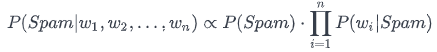The probability of the message containing words (w1, w2, w3,...) being spam is proportional to the probability to get the spam multiplied by a product of probabilities for every word in the message to belong to a spam message. What does that imply? We measure the likelihood of finding spam for each word in the letter. In our context:

• P_spam — the part of spam messages in our dataset
• P_wi_spam — the probability of a word to be found in the spam messages.

The same logic is used to define:

• P_not_spam — the part of non-spam messages in the dataset
• P_wi_non_spam — the probability of a word to be found in the non-spam messages.

But we still do not know how to measure the probability of each word. We do have another formula, though: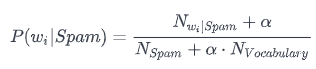What do we have here:

• N_vocabulary — the number of unique words in the whole dataset.
• N_spam — the total number of words in the spam messages.
• N_wi_spam — the number of a word repeats in all spam messages.
• Alpha — the coefficient for the cases when a word in the message is absent in our dataset.

Shortly: a word's likelihood of belonging to a spam message is the frequency of this word in our dataset's "spam part”.

The same formula (but with other values) is also valid for the probability of a word belonging to messages that are not spam.

## Detecting Email Spam

For our purpose, we will use the collection of SMS messages, which was put together by Tiago A. Almeida and José María Gómez Hidalgo. It is free and can be downloaded from the UCI Machine Learning Repository.

The structure of the dataset is straightforward. It includes two columns, one for the "spam/ham" label and another for the message text.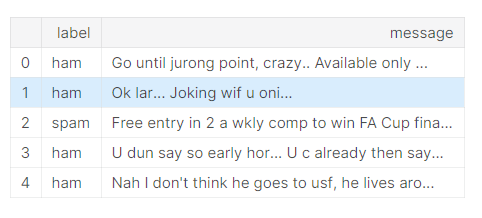### Exploratory Data Analysis (EDA)

``````sms.describe()
``````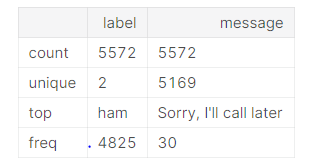``````sms.groupby('label').describe()
``````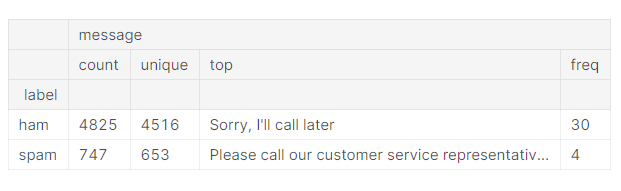We have 4825 ham message and 747 spam message.

Converting label to a numerical variable

``````sms['label_num'] = sms.label.map({'ham':0, 'spam':1})
``````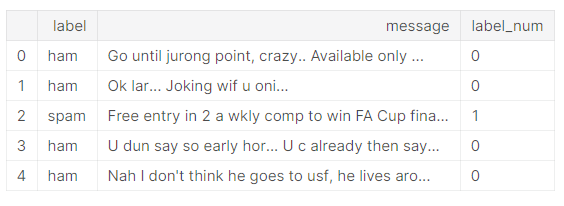As we continue our analysis we want to start thinking about the features we are going to be using. This goes along with the general idea of feature engineering. The better your domain knowledge on the data, the better your ability to engineer more features from it. Feature engineering is a very large part of spam detection in general.

``````sms['message_len'] = sms.message.apply(len)
``````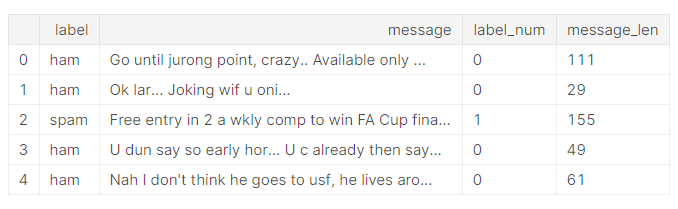``````plt.figure(figsize=(12, 8))

sms[sms.label=='ham'].message_len.plot(bins=35, kind='hist', color='blue', label='Ham messages', alpha=0.6)

sms[sms.label=='spam'].message_len.plot(kind='hist', color='red', label='Spam messages', alpha=0.6)

plt.legend()
plt.xlabel("Message Length")
``````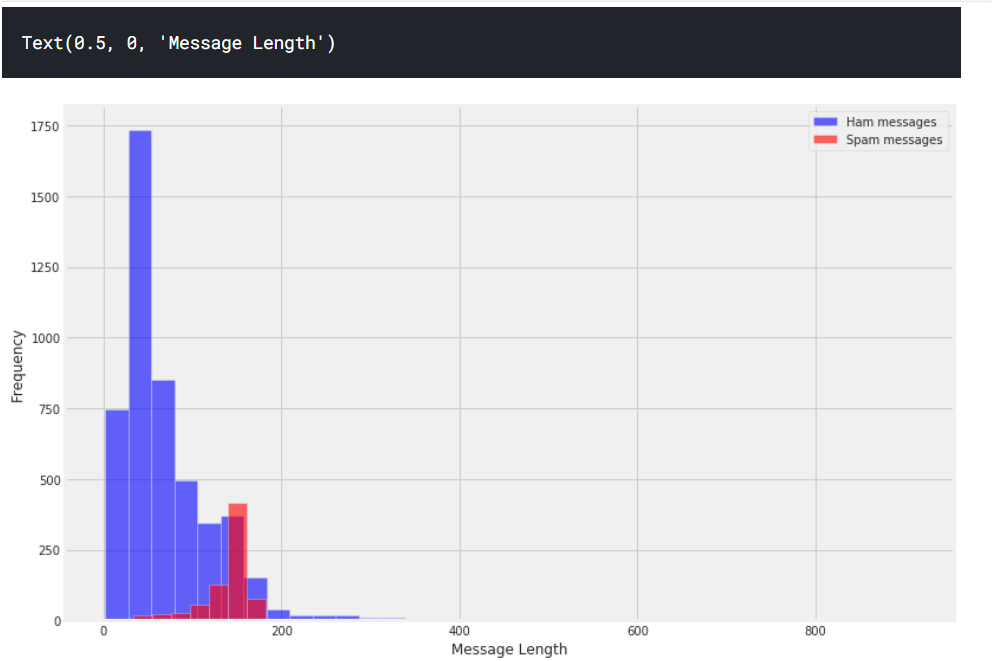Very interesting! Through just basic EDA we've been able to discover a trend that spam messages tend to have more characters.

``````sms[sms.label=='ham'].describe()
``````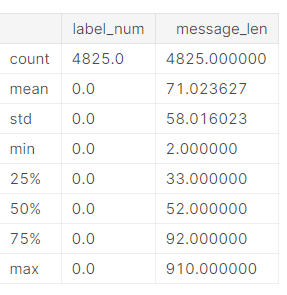``````sms[sms.label=='spam'].describe()
``````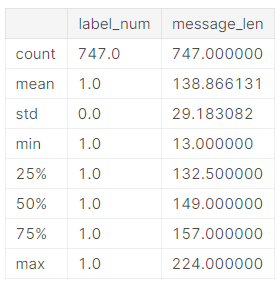### Text Pre-processing

We need to prepare data before the application of the algorithm. We will remove the punctuation first of all. Then all the text will be converted to the lower case and split into separate words.

``````sms_data_clean = sms_data.copy()
``````
``````sms_data_clean['SMS'] = sms_data_clean['SMS'].str.replace('\W+', '').str.replace('\s+', ' ').str.strip()
``````
``````sms_data_clean['SMS'] = sms_data_clean['SMS'].str.lower()
``````
``````sms_data_clean['SMS'] = sms_data_clean['SMS'].str.split()
``````
``````sms_data_clean['SMS'].head()
``````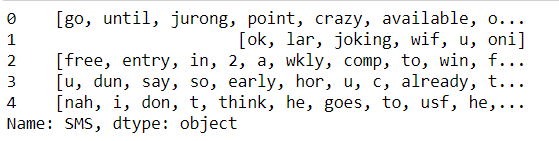``````sms_data_clean['Label'].value_counts() / sms_data.shape*100
``````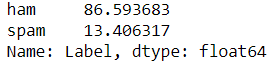We need to split the dataset to train and test data. At the same time we also need to keep the distribution of spam and non-spam messages.

``````train_data = sms_data_clean.sample(frac=0.8,random_state=1).reset_index(drop=True)
test_data = sms_data_clean.drop(train_data.index).reset_index(drop=True)
train_data = train_data.reset_index(drop=True)
``````
``````train_data['Label'].value_counts() / train_data.shape*100
``````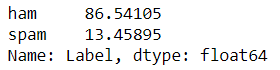``````train_data.shape
``````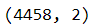``````test_data['Label'].value_counts() / test_data.shape * 100
``````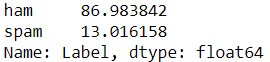``````test_data.shape
``````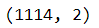``````test_data.head()
``````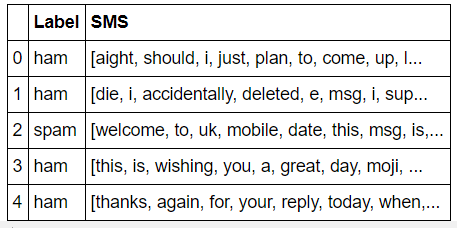And finally, we have to prepare the vocabulary for each message and count the number of separate words in the same.

``````vocabulary = list(set(train_data['SMS'].sum()))
``````
``````vocabulary[11:20]
``````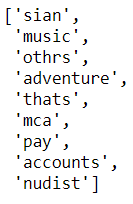``````len(vocabulary)
``````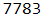#### Calculate frequencies of the words for each message

``````word_counts_per_sms = pd.DataFrame([
[row.count(word) for word in vocabulary]
for _, row in train_data.iterrows()], columns=vocabulary)
``````
``````train_data = pd.concat([train_data.reset_index(), word_counts_per_sms], axis=1).iloc[:,1:]
``````
``````train_data = pd.concat([train_data.reset_index(), word_counts_per_sms], axis=1).iloc[:,1:]
``````
``````train_data.head()
``````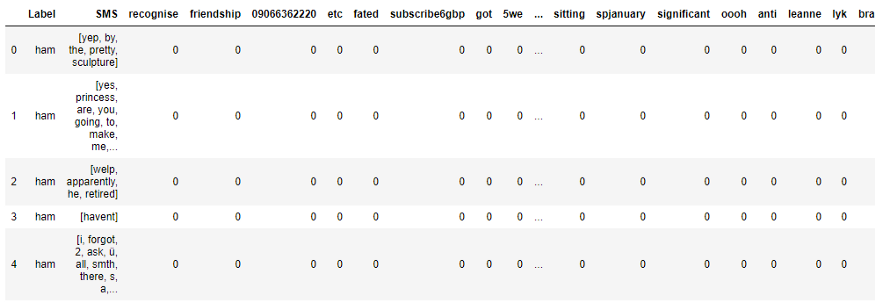## Building and Evaluating The Model

We are going to follow the formulas mentioned above and we will define the main values.

## Calculate values for the Bayes formula

• Probability of message to be Spam
``````Pspam = train_data[‘Label’].value_counts()[‘spam’] / train_data.shape
``````
• Probability of message to be Non-Spam
``````Pham = train_data[‘Label’].value_counts()[‘ham’] / train_data.shape
``````
• Number of words in Spam Messages
``````Nspam = train_data.loc[train_data[‘Label’] == ‘spam’,
‘SMS’].apply(len).sum()
``````
• Number of words in Non-Spam Messages
``````Nham = train_data.loc[train_data[‘Label’] == ‘ham’,
‘SMS’].apply(len).sum()
``````
• Size of the Vocabulary
``````Nvoc = len(train_data.columns - 3)
``````
• Set alpha
``````alpha = 1
``````

To complete the formula we will define the functions and evaluate the probability that the word belongs to spam and non-spam messages:

``````def p_w_spam(word):
if word in train_data.columns:
return (train_data.loc[train_data['Label'] == 'spam', word].sum() + alpha) / (Nspam + alpha*Nvoc)
else:
return 1
``````
``````def p_w_ham(word):
if word in train_data.columns:
return (train_data.loc[train_data['Label'] == 'ham', word].sum() + alpha) / (Nham + alpha*Nvoc)
else:
return 1
``````

Preparing the Classification Function:

``````def classify(message):
p_spam_given_message = Pspam
p_ham_given_message = Pham
for word in message:
p_spam_given_message *= p_w_spam(word)
p_ham_given_message *= p_w_ham(word)
if p_ham_given_message > p_spam_given_message:
return 'ham'
elif p_ham_given_message < p_spam_given_message:
return 'spam'
else:
return 'needs human classification'

``````
``````classify('secret')
``````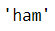``````classify(['secret', 'source', 'of', 'infinite', 'power'])
``````### Use test data

``````test_data['predicted'] = test_data['SMS'].apply(classify)
``````
``````test_data.head()
````````````correct = (test_data['predicted'] == test_data['Label']).sum() / test_data.shape * 100
``````
``````test_data.loc[test_data['predicted'] != test_data['Label']]
``````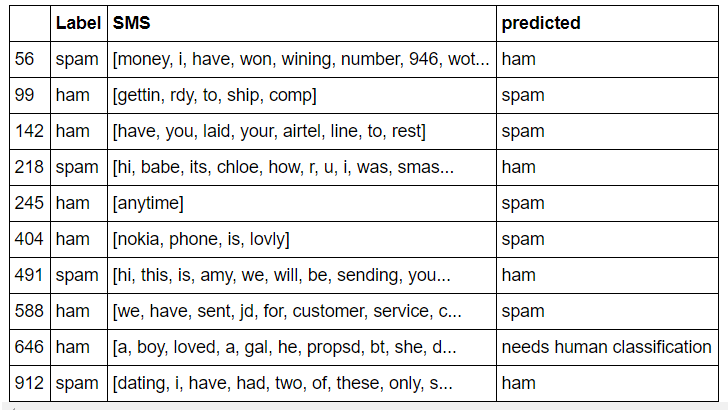``````correct
``````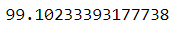### Conclusion

We have acheived an accuracy of 99.1%. It is very impressive as we were able to classify 99.1% of the test data.
Naive Bayes is a strong but simple algorithm that is extremely effective when filtering out spam emails.